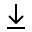Mystery Master Assumptions Member### Introduction

This help article explains what assumptions are. When the Mystery Master solves a logic puzzle, it relies on facts, rules, and laws to find marks. But that may not be enough. When all else fails in finding a solution, the Mystery Master makes assumptions. In other words, it makes a guess. And if the assumption leads to a contradiction, it must undo the assumption, and make another one, until all possibilities have been exhausted. Making assumptions is the last resort of the levels.

Below are the logic puzzles that require assumptions. The Marks column is the number of marks the program enters without assumptions, followed by the total number of marks for the puzzle. The Solution column gives the number of assumptions to find a solution, and the Total column is the total number of assumptions to exhaust all combinations.

Assumptions
Logic Puzzle Marks Solution Total Notes
Modern Novels 59/150 1 2
Six Fearsome Heroes 76/360 11 24
Small Town Motels 719/1215 3 12
The Smith-Jones-Robinson Classic 38/54 1/2 2 2 solutions because clues are ambiguous, but Smith is the engineer in both.
Student Logic 0/16 10 10 Need assumptions since there are no facts.

### Conclusion

It is no coincidence that all of these puzzles have rules. If I wrote better rules, this may diminish/eliminate the number of assumptions.